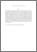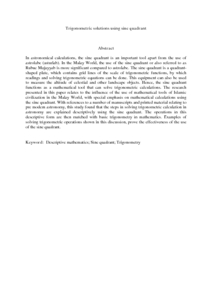# Trigonometric solutions using sine quadrant

## Citation

Zainal, Baharrudin and Ismail, Mat Rofa (2010) Trigonometric solutions using sine quadrant. Procedia - Social and Behavioral Sciences, 8. pp. 721-728. ISSN 1877-0428

## Abstract

In astronomical calculations, the sine quadrant is an important tool apart from the use of astrolabe (asturlab). In the Malay World, the use of the sine quadrant or also referred to as Rubuc Mujayyab is more significant compared to astrolabe. The sine quadrant is a quadrant-shaped plate, which contains grid lines of the scale of trigonometric functions, by which readings and solving trigonometric equations can be done. This equipment can also be used to measure the altitude of celestial and other landscape objects. Hence, the sine quadrant functions as a mathematical tool that can solve trigonometric calculations. The research presented in this paper relates to the influence of the use of mathematical tools of Islamic civilization in the Malay World, with special emphasis on mathematical calculations using the sine quadrant. With references to a number of manuscripts and printed material relating to pre modern astronomy, this study found that the steps in solving trigonometric calculation in astronomy are explained descriptively using the sine quadrant. The operations in this descriptive form are then matched with basic trigonometry in mathematics. Examples of solving trigonometric operations shown in this discussion, prove the effectiveness of the use of the sine quadrant.Preview
PDF (Abstract)View Item